Question

Determine V1, V2 and V3 for the circuit below (assume ideal diodes).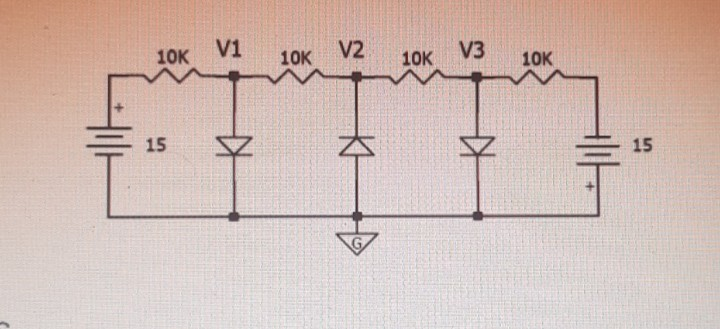We need at least 9 more requests to produce the answer.

1 / 10 have requested this problem solution

The more requests, the faster the answer.

All students who have requested the answer will be notified once they are available.

#### Earn Coins

Coins can be redeemed for fabulous gifts.

Similar Homework Help Questions
• ### In the given circuit, obtain V1, V2, and V3. Assume A = 130 V, B =...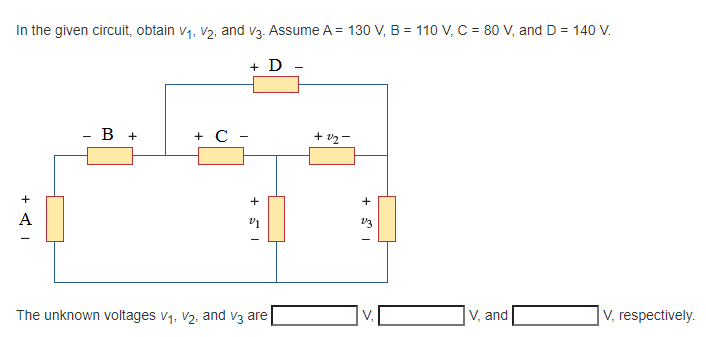In the given circuit, obtain V1, V2, and V3. Assume A = 130 V, B = 110 V, C = 80 V, and D = 140 V. + D - - B + + C- + 12 + + + A V1 13 The unknown voltages V1, V2, and V3 are V V, and V, respectively

• ### Assume V1, V2 and V3 in the circuit below can either indicate 0V or 1V. Therefore,...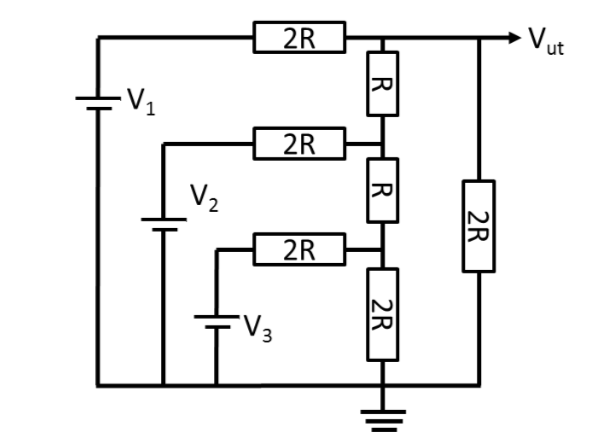Assume V1, V2 and V3 in the circuit below can either indicate 0V or 1V. Therefore, 8 (eight) exist different alternate voltage combinations. Calculate Vut for all cases. ut 1 2R 2R

• ### ideal diodes 2 Assuming ideal diodes, for the diode circuit shown: Select one: a. None of...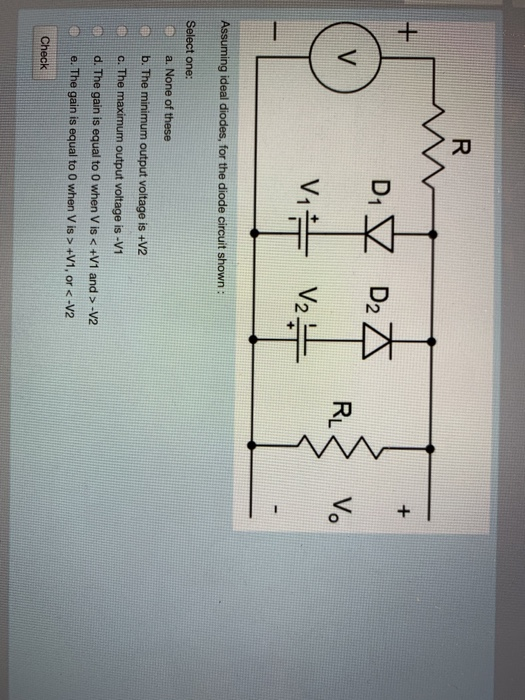ideal diodes 2 Assuming ideal diodes, for the diode circuit shown: Select one: a. None of these b. The minimum output voltage is +V2 c. The maximum output voltage is -V1 d. The gain is equal to 0 when V is < +V1 and > -V2 e. The gain is equal to O when V is > +V1, or < -v2 Ch

• ### Please use nodal analysis to find V1, V2, and V3 Given the circuit which will be...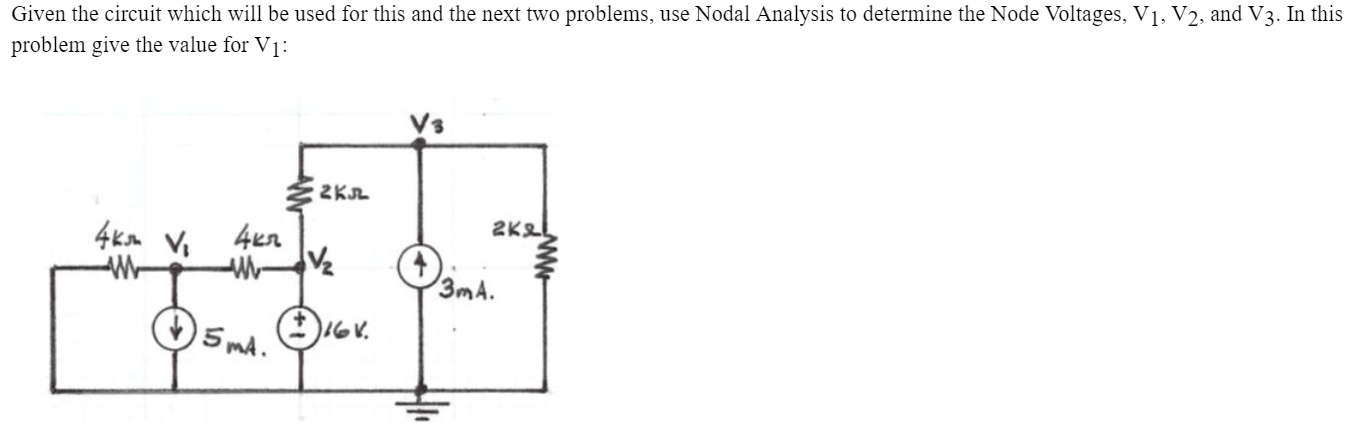Please use nodal analysis to find V1, V2, and V3 Given the circuit which will be used for this and the next two problems, use Nodal Analysis to determine the Node Voltages, V1, V2, and V3. In this problem give the value for V1: 2KR 4ksh Vi 4kr 2KL va 3mA. 15mA. Gr.

• ### uestion 4: For the circuit given below, determine Vi, V2, and V3. V2 V3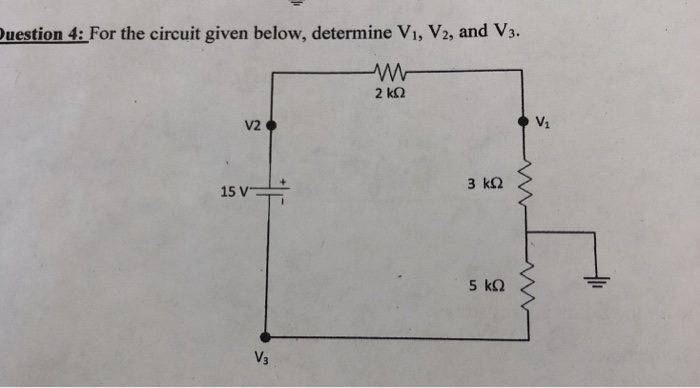uestion 4: For the circuit given below, determine Vi, V2, and V3. V2 V3

• ### To V2 - V4 R1 1 k 2 10 V For the circuit above, assuming ideal...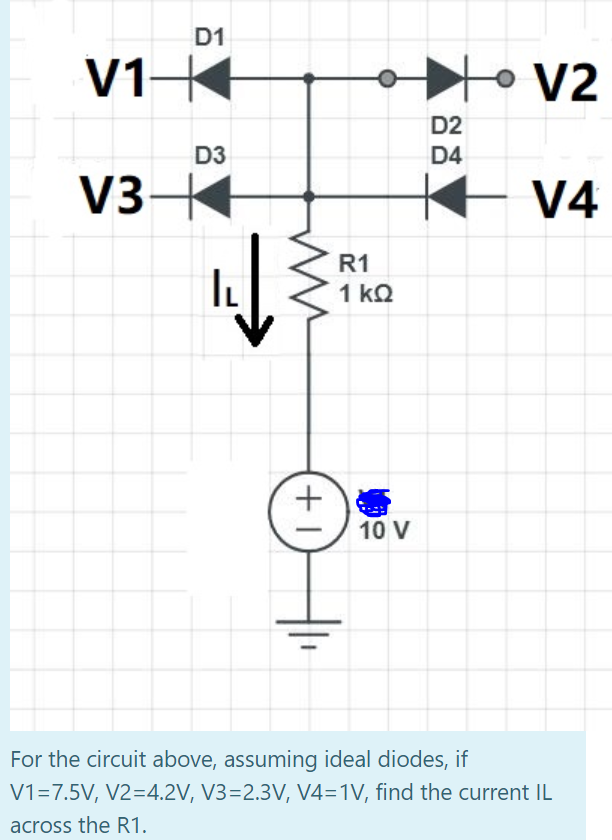To V2 - V4 R1 1 k 2 10 V For the circuit above, assuming ideal diodes, if V1=7.5V, V2=4.2V, V3=2.3V, V4=1V, find the current IL across the R1.

• ### For the given four element circuit loop with voltages V1, V2, V3, then the voltage v4...For the given four element circuit loop with voltages V1, V2, V3, then the voltage v4 is given by: + V₂ V4 = -V1 - V2 - Vz V4 = -V1 + V2 - 13 V4 = V1 + V2 + V3 14 = -V1 + V2 + V3

• ### Find voltages.Determine V1, V2 and V3 for the circuit below (assume ideal diodes).

• ### 1) Determine if w is in the subspace spanned by v1, v2, v3 2) Are the...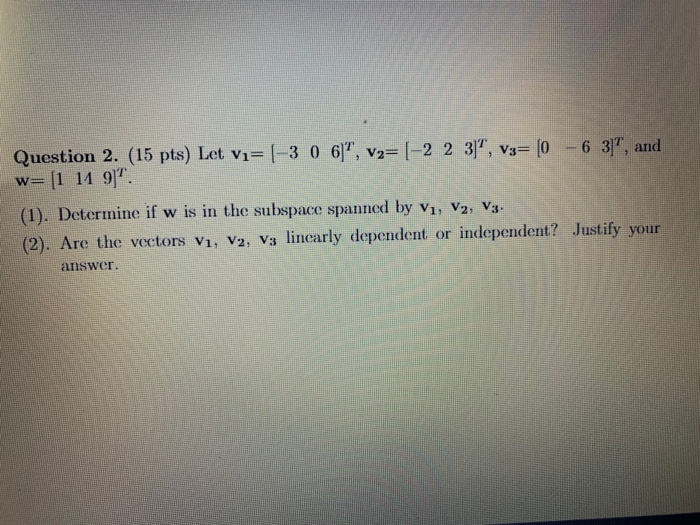1) Determine if w is in the subspace spanned by v1, v2, v3 2) Are the vectors v1, v2, v3 linearly dependent or independent? justify your answer Question 2. (15 pts) Let vi=(-3 0 6)", v2= (-2 2 3]", V3= (0 - 6 37, and w= [1 11 9". (1). Determine if w is in the subspace spanned by V1, V2, V3. (2). Are the vectors V1, V2, V3 linearly dependent or independent? Justify your answer

• ### 6) Assume ideal op-amps. Write the voltage node equations for the following circuit Rb V3 Ra...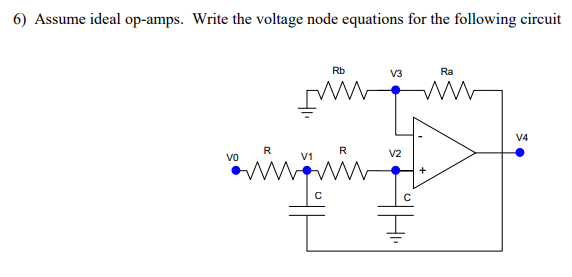6) Assume ideal op-amps. Write the voltage node equations for the following circuit Rb V3 Ra VA VO V1 V2